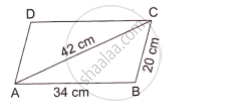# The Adjacent Sides of a Parallelogram Abcd Measure 34 Cm and 20 Cm, and the Diagonal Ac Measures 42 Cm. Find the Area of the Parallelogram. - Mathematics

The adjacent sides of a parallelogram ABCD measure 34 cm and 20 cm, and the diagonal AC measures 42 cm. Find the area of the parallelogram.#### Solution

Parallelogram ABCD is made up of congruent ΔABC and ΔADC

Area of triangle ABC= sqrt (s (s-a)(s-b)(s-c))

Thus, we have :

s=(a+b+c)/2

s=(34+20+42)/2

s=48 cm

Area of Δ ABC=sqrt(48(48-34)(48-20)(48-42))

=sqrt(48xx14xx28xx6)

=336 cm^2

Now,

Area of the parallelogram=2xx Area of ΔABC

=2xx336

=672 cm^2

Concept: Circumference of a Circle
Is there an error in this question or solution?

#### APPEARS IN

RS Aggarwal Secondary School Class 10 Maths
Chapter 17 Perimeter and Areas of Plane Figures
Q 31